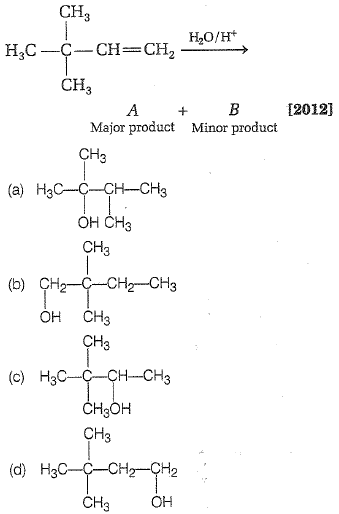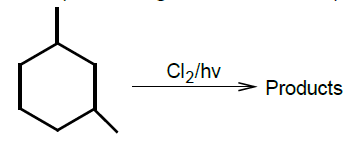In the following reaction,Concept Questions :-

Alkanes, Alkenes and Alkynes - Chemical Properties
High Yielding Test Series + Question Bank - NEET 2020

Difficulty Level:

CH3-C$\equiv$C-H$\stackrel{{\mathrm{CH}}_{3}\mathrm{MgBr}}{\to }$CH4 + (A)(B); (B) will be

1. H3C-CH2-CH2-COOH                                2. CH3-C$\equiv$C-CH3

3. H3C-C$\equiv$C-COOH                                     4. H3C-CH=CH-COOH

Concept Questions :-

Alkanes, Alkenes and Alkynes - Chemical Properties
High Yielding Test Series + Question Bank - NEET 2020

Difficulty Level:

The monochlorinated products (excluding stereo-isomers) obtained from the reaction is:(1) 4            (2) 5              (3) 6            (4) 7

Concept Questions :-

Alkanes, Alkenes and Alkynes - Chemical Properties
High Yielding Test Series + Question Bank - NEET 2020

Difficulty Level:

3-hexyne can be converted to trans-3-hexene by the action of:

1. ${\mathrm{H}}_{2}$ - Pd/ ${\mathrm{BaSO}}_{4}$                       2. Li-Liq. ${\mathrm{NH}}_{3}$

3. ${\mathrm{H}}_{2}$ - Pt ${\mathrm{O}}_{2}$                                4. ${\mathrm{NaBH}}_{4}$

Concept Questions :-

Alkanes, Alkenes and Alkynes -Methods of Preparation
High Yielding Test Series + Question Bank - NEET 2020

Difficulty Level:

Which one of the following has the shortest carbon-carbon bond length?

1. Benzene                                  2. Ethene

3. Ethyne                                    4. Ethane

Concept Questions :-

Aliphatic Hydrocarbon- physical properties
High Yielding Test Series + Question Bank - NEET 2020

Difficulty Level:

Reaction of HBr with propene in the presence of peroxide gives

1. iso-propyl bromide

2. 3-bromo propane

3. allyl bromide

4. n-propyl bromide

High Yielding Test Series + Question Bank - NEET 2020

Difficulty Level:

In Friedel-Craft's synthesis of toluene, the reactants in addition to anhydrous ${\mathrm{AlCl}}_{3}$ are

(a)

(b) ${\mathrm{C}}_{6}{\mathrm{H}}_{5}\mathrm{Cl}$ + ${\mathrm{CH}}_{3}\mathrm{Cl}$

(c) ${\mathrm{C}}_{6}{\mathrm{H}}_{6}$ + ${\mathrm{CH}}_{4}$

(d) ${\mathrm{C}}_{6}{\mathrm{H}}_{6}$ + ${\mathrm{CH}}_{3}\mathrm{Cl}$

Concept Questions :-

Aromatic Hydrocarbons - Benzene - Structure, Preparation & Chemical Reactions
High Yielding Test Series + Question Bank - NEET 2020

Difficulty Level:

The reaction of toluene with ${\mathrm{Cl}}_{2}$ in the presence of ${\mathrm{FeCl}}_{3}$ gives 'X' and reaction in presence of light gives 'Y'. Thus, 'X' and  'Y' are

1. X = benzal chloride, Y= o-chlorotoluene

2. X = m-chlorotoluene, Y= p-chlorotoluene

3. X = o and p-chlorotoluene, Y = trichloromethyl benzene

4. X = benzal chloride, Y = m-chlorotoluene

Concept Questions :-

Aromatic Hydrocarbons - Functional Groups, Directive Influence in Monosubstituted Benzene
High Yielding Test Series + Question Bank - NEET 2020

Difficulty Level:

In the reaction, ${\mathrm{C}}_{6}{\mathrm{H}}_{5}{\mathrm{CH}}_{3}\stackrel{\mathrm{Oxidation}}{\to }$$\stackrel{\mathrm{NaOH}}{\to }$B

the product C is:

1. ${\mathrm{C}}_{6}{\mathrm{H}}_{5}\mathrm{OH}$                              2. ${\mathrm{C}}_{6}{\mathrm{H}}_{6}$

3. ${\mathrm{C}}_{6}{\mathrm{H}}_{5}\mathrm{COONa}$                        4. ${\mathrm{C}}_{6}{\mathrm{H}}_{5}\mathrm{ONa}$

Concept Questions :-

Aromatic Hydrocarbons - Benzene - Structure, Preparation & Chemical Reactions
High Yielding Test Series + Question Bank - NEET 2020

Difficulty Level:

When acetylene is passed through dil. ${\mathrm{H}}_{2}{\mathrm{SO}}_{4}$ in presence of ${\mathrm{HgSO}}_{4}$, the compound formed is

1. ether                                    2. ketone

3. acetic acid                             4. acetaldehyde

Concept Questions :-

Alkanes, Alkenes and Alkynes - Chemical Properties